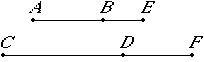# Proposition 105

A straight line commensurable with a minor straight line is minor.

Let AB be a minor straight line, and CD commensurable with AB.

I say that CD is also minor.Make the same construction as before. Then, since AE and EB are incommensurable in square, therefore CF and FD are also incommensurable in square.

Now since AE is to EB as CF is to FD, therefore the square on AE is to the square on EB as the square on CF is to the square on FD.

V.18

Therefore, taken jointly, the sum of the squares on AE and EB is to the square on EB as the sum of the squares on CF and FD is to the square on FD.

But the square on BE is commensurable with the square on DF, therefore the sum of the squares on AE and EB is also commensurable with the sum of the squares on CF and FD.

But the sum of the squares on AE and EB is rational, therefore the sum of the squares on CF and FD is also rational.

Again, since the square on AE is to the rectangle AE by EB as the square on CF is to the rectangle CF by FD, while the square on AE is commensurable with the square on CF, therefore the rectangle AE by EB is also commensurable with the rectangle CF by FD.

But the rectangle AE by EB is medial, therefore the rectangle CF by FD is also medial.

Therefore CF and FD are straight lines incommensurable in square which make the sum of the squares on them rational, but the rectangle contained by them medial.

Therefore CD is minor.

X.76

Therefore, a straight line commensurable with a minor straight line is minor.

Q.E.D.

## Guide

This proposition is not used in the rest of the Elements.